StatLect

# Information matrix

The information matrix (also called Fisher information matrix) is the matrix of second cross-moments of the score vector. The latter is the vector of first partial derivatives of the log-likelihood function with respect to its parameters.## Definition

The information matrix is defined as follows.

Definition Letbe aparameter vector characterizing the distribution of a sample. Letbe the likelihood function of, depending on the parameter. Letbe the log-likelihood functionDenote bythe score vector, that is, thevector of first derivatives ofwith respect to the entries of. The information matrixis thematrix of second cross-moments of the score, defined bywhere the notationindicates that the expected value is taken with respect to the probability distribution associated to the parameter.

For example, if the samplehas a continuous distribution, then the likelihood function iswhereis the probability density function of, parametrized by, and the information matrix is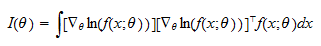## The information matrix is the covariance matrix of the score

Under mild regularity conditions, the expected value of the score is equal to zero:As a consequence,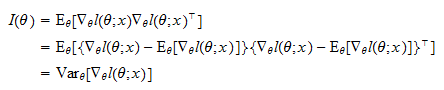that is, the information matrix is the covariance matrix of the score.

## Information equality

Under mild regularity conditions, it can be proved thatwhereis the matrix of second-order cross-partial derivatives (so-called Hessian matrix) of the log-likelihood.

This equality is called information equality.

## Information matrix of the normal distribution

As an example, consider a samplemade up of the realizations ofIID normal random variables with parametersand(mean and variance).

In this case, the information matrix isProof

The log-likelihood function is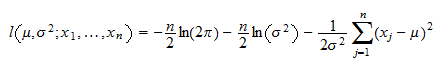as proved in the lecture on maximum likelihood estimation of the parameters of the normal distribution. The scoreis avector whose entries are the partial derivatives of the log-likelihood with respect toand: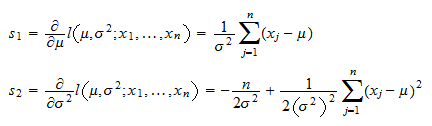The information matrix is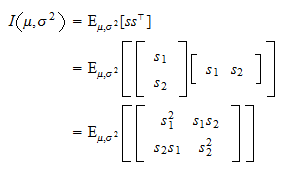We havewhere: in stepwe have used the fact that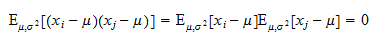forbecause the variables in the sample are independent and have mean equal to; in stepwe have used the fact thatMoreover,where: in stepsandwe have used the independence of the observations in the sample and in stepwe have used the fact that the fourth central moment of the normal distribution is equal to. Finally,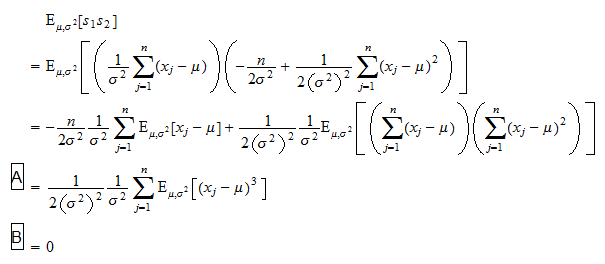where: in stepwe have used the facts thatand that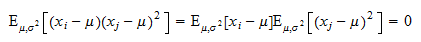forbecause the variables in the sample are independent; in stepwe have used the fact that the third central moment of the normal distribution is equal to zero.

## More details

More details about the Fisher information matrix, including proofs of the information equality and of the fact that the expected value of the score is equal to zero, can be found in the lecture entitled Maximum likelihood.